Anzeige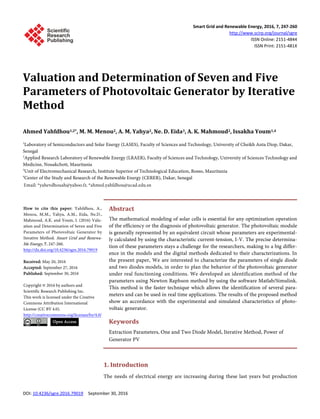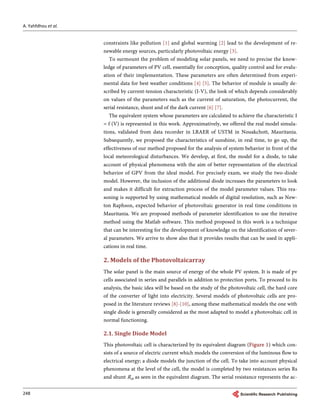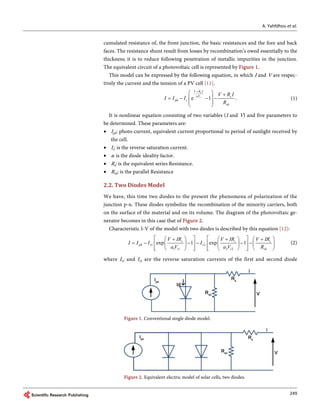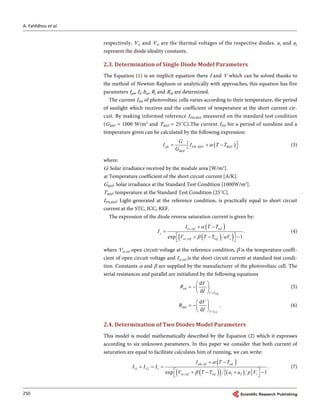Anzeige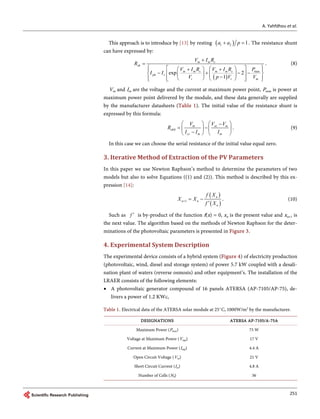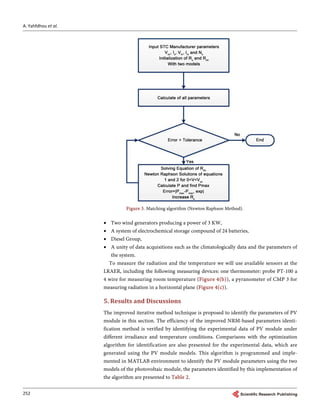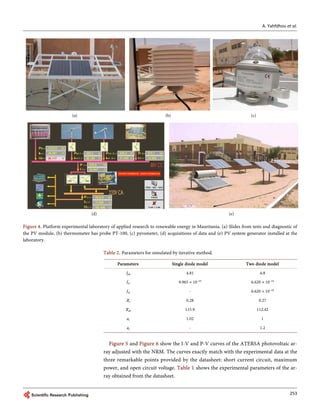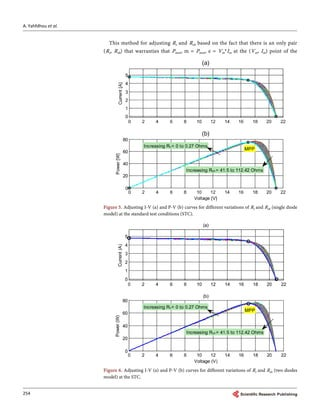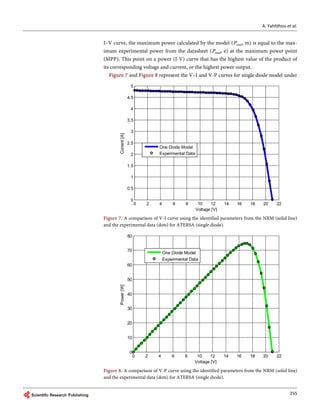Anzeige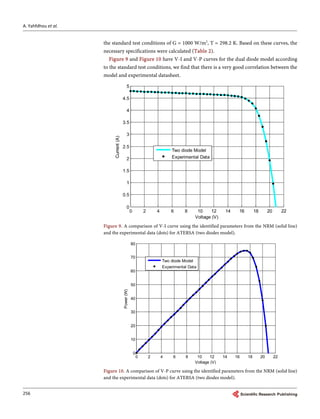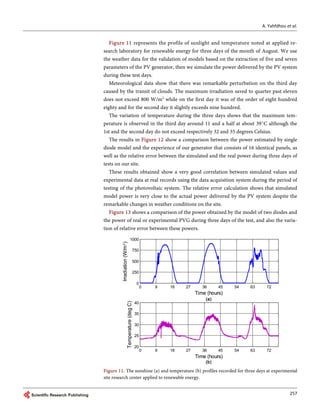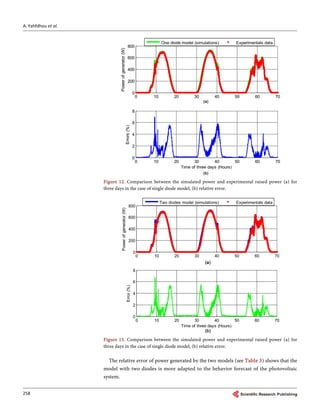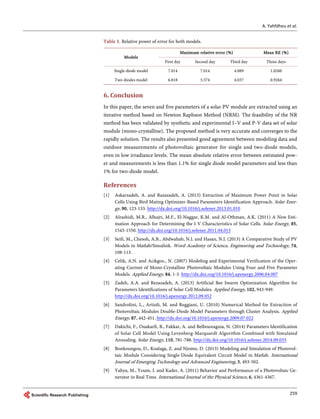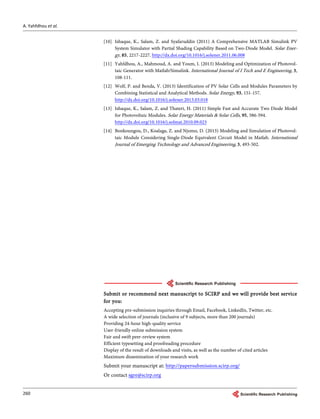Nächste SlideShare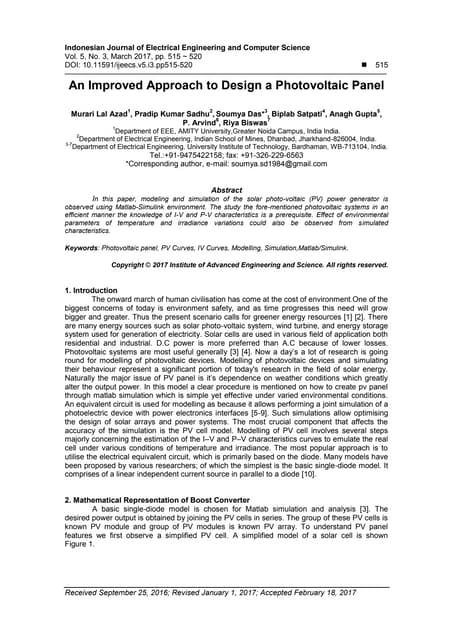06 5 feb17 13807 an improved soumya(edit)
1 von 14
Anzeige

### Valuation and Determination of Seven and Five Parameters of Photovoltaic Generator by Iterative Method

2. A. Yahfdhou et al. 248 constraints like pollution  and global warming  lead to the development of re- newable energy sources, particularly photovoltaic energy . To surmount the problem of modeling solar panels, we need to precise the know- ledge of parameters of PV cell, essentially for conception, quality control and for evalu- ation of their implementation. These parameters are often determined from experi- mental data for best weather conditions  . The behavior of module is usually de- scribed by current-tension characteristic (I-V), the look of which depends considerably on values of the parameters such as the current of saturation, the photocurrent, the serial resistance, shunt and of the dark current  . The equivalent system whose parameters are calculated to achieve the characteristic I = f (V) is represented in this work. Approximatively, we offered the real model simula- tions, validated from data recorder in LRAER of USTM in Nouakchott, Mauritania. Subsequently, we proposed the characteristics of sunshine, in real time, to go up, the effectiveness of our method proposed for the analysis of system behavior in front of the local meteorological disturbances. We develop, at first, the model for a diode, to take account of physical phenomena with the aim of better representation of the electrical behavior of GPV from the ideal model. For precisely exam, we study the two-diode model. However, the inclusion of the additional diode increases the parameters to look and makes it difficult for extraction process of the model parameter values. This rea- soning is supported by using mathematical models of digital resolution, such as New- ton Raphson, expected behavior of photovoltaic generator in real time conditions in Mauritania. We are proposed methods of parameter identification to use the iterative method using the Matlab software. This method proposed in this work is a technique that can be interesting for the development of knowledge on the identification of sever- al parameters. We arrive to show also that it provides results that can be used in appli- cations in real time. 2. Models of the Photovoltaicarray The solar panel is the main source of energy of the whole PV system. It is made of pv cells associated in series and parallels in addition to protection ports. To proceed to its analysis, the basic idea will be based on the study of the photovoltaic cell, the hard core of the converter of light into electricity. Several models of photovoltaic cells are pro- posed in the literature reviews -, among these mathematical models the one with single diode is generally considered as the most adapted to model a photovoltaic cell in normal functioning. 2.1. Single Diode Model This photovoltaic cell is characterized by its equivalent diagram (Figure 1) which con- sists of a source of electric current which models the conversion of the luminous flow to electrical energy; a diode models the junction of the cell. To take into account physical phenomena at the level of the cell, the model is completed by two resistances series Rs and shunt Rsh as seen in the equivalent diagram. The serial resistance represents the ac-
3. A. Yahfdhou et al. 249 cumulated resistance of, the front junction, the basic resistances and the fore and back faces. The resistance shunt result from losses by recombination’s owed essentially to the thickness; it is to reduce following penetration of metallic impurities in the junction. The equivalent circuit of a photovoltaic cell is represented by Figure 1. This model can be expressed by the following equation, in which I and V are respec- tively the current and the tension of a PV cell . e 1 s t V R I aV s ph s sh V R I I I I R +   +  = − − −     . (1) It is nonlinear equation consisting of two variables (I and V) and five parameters to be determined. These parameters are: • Iph: photo-current, equivalent current proportional to period of sunlight received by the cell. • Is: is the reverse saturation current. • a: is the diode ideality factor. • Rs: is the equivalent series Resistance. • Rsh: is the parallel Resistance 2.2. Two Diodes Model We have, this time two diodes to the present the phenomena of polarization of the junction p-n. These diodes symbolize the recombination of the minority carriers, both on the surface of the material and on its volume. The diagram of the photovoltaic ge- nerator becomes in this case that of Figure 2. Characteristic I-V of the model with two diodes is described by this equation : 1 2 1 1 2 2 exp 1 exp 1s s s ph s s t t sh V IR V IR V IR I I I I a V a V R         + + + = − − − − −                     (2) where Is1 and Is2 are the reverse saturation currents of the first and second diode Figure 1. Conventional single diode model. Figure 2. Equivalent electric model of solar cells, two diodes.
4. A. Yahfdhou et al. 250 respectively, Vt1 and Vt2 are the thermal voltages of the respective diodes. a1 and a2 represent the diode ideality constants. 2.3. Determination of Single Diode Model Parameters The Equation (1) is an implicit equation there I and V which can be solved thanks to the method of Newton-Raphson or analytically with approaches, this equation has five parameters Iph, Is, has, Rs and Rsh are determined. The current IPH of photovoltaic cells varies according to their temperature, the period of sunlight which receives and the coefficient of temperature at the short current cir- cuit. By making informed reference IPH,REF measured on the standard test condition (GREF = 1000 W/m2 and TREF = 25˚C).The current IPH for a period of sunshine and a temperature given can be calculated by the following expression: ( ),ph PH REF REF REF G I I T T G α = + −  (3) where: G: Solar irradiance received by the module area [W/m2 ]. α: Temperature coefficient of the short circuit current [A/K]. GREF: Solar irradiance at the Standard Test Condition [1000W/m2 ]. TREF: temperature at the Standard Test Condition [25˚C]. IPH,REF: Light-generated at the reference condition, is practically equal to short circuit current at the STC, ICC, REF. The expression of the diode reverse saturation current is given by: ( ) ( )( ) , ,exp 1 cc ref ref s oc ref ref t I T T I V T T aV α β + − =  + − −   . (4) where Voc,ref open circuit voltage at the reference condition, β is the temperature coeffi- cient of open circuit voltage and Icc,ref is the short circuit current at standard test condi- tion. Constants α and β are supplied by the manufacturer of the photovoltaic cell. The serial resistances and parallel are initialized by the following equations 0 d d OC s V V V R I =   = −    (5) 0 d d CC sh I I V R I =   = −    . (6) 2.4. Determination of Two Diodes Model Parameters This model is model mathematically described by the Equation (2) which it expresses according to six unknown parameters. In this paper we consider that both current of saturation are equal to facilitate calculates him of running, we can write: ( ) ( )( ) ( ){ } , 1 2 , 1 2exp 1 ph ref ref s s s oc ref ref t I T T I I I V T T a a p V α β + − = = =  + − + −   . (7)
5. A. Yahfdhou et al. 251 This approach is to introduce by  by resting ( )1 2 1a a p+ =. The resistance shunt can have expressed by: ( ) max exp 2 1 m m s sh m m s m m s ph s t t m V I R R V I R V I R P I I V p V V + =     + +  − + − −      −        . (8) Vm and Im are the voltage and the current at maximum power point, Pmax is power at maximum power point delivered by the module, and these data generally are supplied by the manufacturer datasheets (Table 1). The initial value of the resistance shunt is expressed by this formula: 0 m oc m sh cc m m V V V R I I I    − = −    −    . (9) In this case we can choose the serial resistance of the initial value equal zero. 3. Iterative Method of Extraction of the PV Parameters In this paper we use Newton Raphson’s method to determine the parameters of two models but also to solve Equations ((1) and (2)). This method is described by this ex- pression : ( ) ( )1 n n n n f X X X f X += − ′ . (10) Such as f ′ is by-product of the function f(x) = 0, xn is the present value and xn+1 is the next value. The algorithm based on the methods of Newton Raphson for the deter- minations of the photovoltaic parameters is presented in Figure 3. 4. Experimental System Description The experimental device consists of a hybrid system (Figure 4) of electricity production (photovoltaic, wind, diesel and storage system) of power 5.7 kW coupled with a desali- nation plant of waters (reverse osmosis) and other equipment’s. The installation of the LRAER consists of the following elements: • A photovoltaic generator compound of 16 panels ATERSA (AP-7105/AP-75), de- livers a power of 1.2 KWc, Table 1. Electrical data of the ATERSA solar module at 25˚C, 1000W/m2 by the manufacturer. DESIGNATIONS ATERSA AP-7105/A-75A Maximum Power (Pmax) 75 W Voltage at Maximum Power (Vmp) 17 V Current at Maximum Power (Imp) 4.4 A Open Circuit Voltage (Voc) 21 V Short Circuit Current (Isc) 4.8 A Number of Cells (NS) 36
6. A. Yahfdhou et al. 252 Figure 3. Matching algorithm (Newton Raphson Method). • Two wind generators producing a power of 3 KW, • A system of electrochemical storage compound of 24 batteries, • Diesel Group, • A unity of data acquisitions such as the climatologically data and the parameters of the system. To measure the radiation and the temperature we will use available sensors at the LRAER, including the following measuring devices: one thermometer: probe PT-100 a 4 wire for measuring room temperature (Figure 4(b)), a pyranometer of CMP 3 for measuring radiation in a horizontal plane (Figure 4(c)). 5. Results and Discussions The improved iterative method technique is proposed to identify the parameters of PV module in this section. The efficiency of the improved NRM-based parameters identi- fication method is verified by identifying the experimental data of PV module under different irradiance and temperature conditions. Comparisons with the optimization algorithm for identification are also presented for the experimental data, which are generated using the PV module models. This algorithm is programmed and imple- mented in MATLAB environment to identify the PV module parameters using the two models of the photovoltaic module, the parameters identified by this implementation of the algorithm are presented to Table 2.
7. A. Yahfdhou et al. 253 (a) (b) (c) (d) (e) Figure 4. Platform experimental laboratory of applied research to renewable energy in Mauritania. (a) Slides from tests and diagnostic of the PV module, (b) thermometer has probe PT-100, (c) pyrometer, (d) acquisitions of data and (e) PV system generator installed at the laboratory. Table 2. Parameters for simulated by iterative method. Parameters Single diode model Two diode model Iph 4.81 4.8 Is1 9.965 × 10−10 6.620 × 10−10 Is2 - 6.620 × 10−10 Rs 0.28 0.27 Rsh 115.9 112.42 a1 1.02 1 a2 - 1.2 Figure 5 and Figure 6 show the I-V and P-V curves of the ATERSA photovoltaic ar- ray adjusted with the NRM. The curves exactly match with the experimental data at the three remarkable points provided by the datasheet: short current circuit, maximum power, and open circuit voltage. Table 1 shows the experimental parameters of the ar- ray obtained from the datasheet.
8. A. Yahfdhou et al. 254 This method for adjusting Rs and Rsh based on the fact that there is an only pair (Rs, Rsh) that warranties that Pmax, m = Pmax, e = Vm*Im at the (Vm, Im) point of the Figure 5. Adjusting I-V (a) and P-V (b) curves for different variations of Rs and Rsh (single diode model) at the standard test conditions (STC). Figure 6. Adjusting I-V (a) and P-V (b) curves for different variations of Rs and Rsh (two diodes model) at the STC.
9. A. Yahfdhou et al. 255 I–V curve, the maximum power calculated by the model (Pmax, m) is equal to the max- imum experimental power from the datasheet (Pmax, e) at the maximum power point (MPP), This point on a power (I-V) curve that has the highest value of the product of its corresponding voltage and current, or the highest power output. Figure 7 and Figure 8 represent the V–I and V-P curves for single diode model under Figure 7. A comparison of V-I curve using the identified parameters from the NRM (solid line) and the experimental data (dots) for ATERSA (single diode). Figure 8. A comparison of V-P curve using the identified parameters from the NRM (solid line) and the experimental data (dots) for ATERSA (single diode).
10. A. Yahfdhou et al. 256 the standard test conditions of G = 1000 W/m2 , T = 298.2 K. Based on these curves, the necessary specifications were calculated (Table 2). Figure 9 and Figure 10 have V-I and V-P curves for the dual diode model according to the standard test conditions, we find that there is a very good correlation between the model and experimental datasheet. Figure 9. A comparison of V-I curve using the identified parameters from the NRM (solid line) and the experimental data (dots) for ATERSA (two diodes model). Figure 10. A comparison of V-P curve using the identified parameters from the NRM (solid line) and the experimental data (dots) for ATERSA (two diodes model).
11. A. Yahfdhou et al. 257 Figure 11 represents the profile of sunlight and temperature noted at applied re- search laboratory for renewable energy for three days of the month of August. We use the weather data for the validation of models based on the extraction of five and seven parameters of the PV generator, then we simulate the power delivered by the PV system during these test days. Meteorological data show that there was remarkable perturbation on the third day caused by the transit of clouds. The maximum irradiation saved to quarter past eleven does not exceed 800 W/m2 while on the first day it was of the order of eight hundred eighty and for the second day it slightly exceeds nine hundred. The variation of temperature during the three days shows that the maximum tem- perature is observed in the third day around 11 and a half at about 39˚C although the 1st and the second day do not exceed respectively 32 and 35 degrees Celsius. The results in Figure 12 show a comparison between the power estimated by single diode model and the experience of our generator that consists of 16 identical panels, as well as the relative error between the simulated and the real power during three days of tests on our site. These results obtained show a very good correlation between simulated values and experimental data at real records using the data acquisition system during the period of testing of the photovoltaic system. The relative error calculation shows that simulated model power is very close to the actual power delivered by the PV system despite the remarkable changes in weather conditions on the site. Figure 13 shows a comparison of the power obtained by the model of two diodes and the power of real or experimental PVG during three days of the test, and also the varia- tion of relative error between these powers. Figure 11. The sunshine (a) and temperature (b) profiles recorded for three days at experimental site research center applied to renewable energy.
12. A. Yahfdhou et al. 258 Figure 12. Comparison between the simulated power and experimental raised power (a) for three days in the case of single diode model, (b) relative error. Figure 13. Comparison between the simulated power and experimental raised power (a) for three days in the case of single diode model, (b) relative error. The relative error of power generated by the two models (see Table 3) shows that the model with two diodes is more adapted to the behavior forecast of the photovoltaic system.
13. A. Yahfdhou et al. 259 Table 3. Relative power of error for both models. Models Maximum relative error (%) Mean RE (%) First day Second day Third day Three days Single diode model 7.014 7.014 4.089 1.0260 Two diodes model 6.818 5.574 4.037 0.9264 6. Conclusion In this paper, the seven and five parameters of a solar PV module are extracted using an iterative method based on Newton Raphson Method (NRM). The feasibility of the NR method has been validated by synthetic and experimental I–V and P-V data set of solar module (mono-crystalline). The proposed method is very accurate and converges to the rapidly solution. The results also presented good agreement between modeling data and outdoor measurements of photovoltaic generator for single and two-diode models, even in low irradiance levels. The mean absolute relative error between estimated pow- er and measurements is less than 1.1% for single diode model parameters and less than 1% for two-diode model. References  Askarzadeh, A. and Razazadeh, A. (2013) Extraction of Maximum Power Point in Solar Cells Using Bird Mating Optimizer-Based Parameters Identification Approach. Solar Ener- gy, 90, 123-133. http://dx.doi.org/10.1016/j.solener.2013.01.010  Alrashidi, M.R., Alhajri, M.F., El-Naggar, K.M. and Al-Othman, A.K. (2011) A New Esti- mation Approach for Determining the I-V Characteristics of Solar Cells. Solar Energy, 85, 1543-1550. http://dx.doi.org/10.1016/j.solener.2011.04.013  Seifi, M., Chesoh, A.B., Abdwahab, N.I. and Hasan, N.I. (2013) A Comparative Study of PV Models in Matlab/Simulink. Word Academy of Science, Engineering and Technology, 74, 108-113.  Celik, A.N. and Acikgoz., N. (2007) Modeling and Experimental Verification of the Oper- ating Current of Mono-Crystalline Photovoltaic Modules Using Four and Five Parameter Models. Applied Energy, 84, 1-5. http://dx.doi.org/10.1016/j.apenergy.2006.04.007  Zadeh, A.A. and Rezazadeh, A. (2013) Artificial Bee Swarm Optimization Algorithm for Parameters Identifications of Solar Cell Modules. Applied Energy, 102, 943-949. http://dx.doi.org/10.1016/j.apenergy.2012.09.052  Sandrolini, L., Artioli, M. and Reggiani, U. (2010) Numerical Method for Extraction of Photovoltaic Modules Double-Diode Model Parameters through Cluster Analysis. Applied Energy, 87, 442-451. http://dx.doi.org/10.1016/j.apenergy.2009.07.022  Dakichi, F., Ouakarfi, B., Fakkar, A. and Belbounaguia, N. (2014) Parameters Identification of Solar Cell Model Using Levenberg-Marquardt Algorithm Combined with Simulated Annealing. Solar Energy, 110, 781-788. http://dx.doi.org/10.1016/j.solener.2014.09.033  Bonkoungou, D., Koalaga, Z. and Njomo, D. (2013) Modeling and Simulation of Photovol- taic Module Considering Single-Diode Equivalent Circuit Model in Matlab. International Journal of Emerging Technology and Advanced Engineering, 3, 493-502.  Yahya, M., Youm, I. and Kader, A. (2011) Behavior and Performance of a Photovoltaic Ge- nerator in Real Time. International Journal of the Physical Science, 6, 4361-4367.